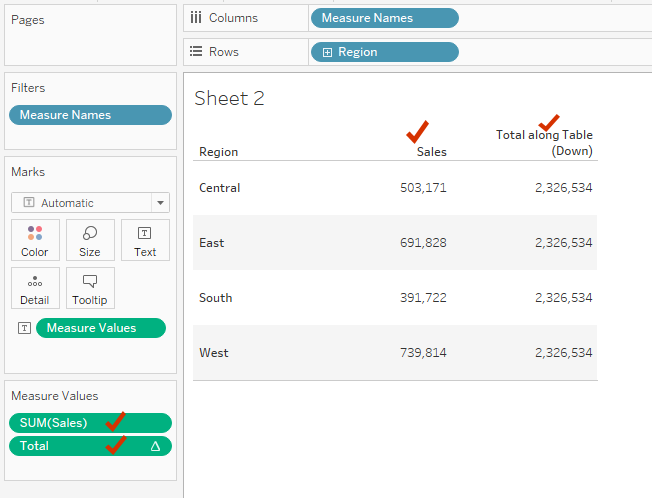top of page
Search
• Bernard K

# SUM () and TOTAL () functions in TableauWhat is the difference between SUM and TOTAL functions in Tableau?

SUM – is an aggregate function used to sum all the values in an expression. Note, SUM can be used with numeric fields ONLY.

Syntax: SUM(<Value>)

TOTAL – is a table calculation function that returns the total of a given expression. (Takes whatever expression you give it and computes the total for the whole data in the partition). Note, TOTAL is not limited to numeric fields like in the case of SUM function.

Syntax: TOTAL(SUM(<Value>)) (Note you can use different aggregate other than SUM)

### Example

Looking at the view below, the SUM function computes the total sales for each Region (sums all sales per region), while the TOTAL function sums all sales for the entire partition (entire data).

Where Total = TOTAL(SUM([Sales]))(Note, TOTAL being a table calculation function, you can customize how the calculation is executed - which in certain cases (based on the direction of computation) will result to the same value as the SUM function).Note adding SUM(Sales) and TOTAL(SUM(Sales)) to a view which is not segmented by any dimension - results to the same value (as the two functions are computed at data source level).### Using TOTAL function to compute sample size in survey analysis

When analyzing survey data, the TOTAL function becomes even more helpful in computing the sample size of your survey. For instance, using the calculation below – where Respondent_ID is the unique identifier of each respondent in your data, you can compute the sample size (total number of respondents) for each survey question as well as for the entire survey as follows.